## The Method of Partial Fractions

Consider the Laplace transform: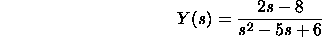Some manipulations must be done before Y(s) can be inverted since it
does not appear directly in our table of Laplace transforms.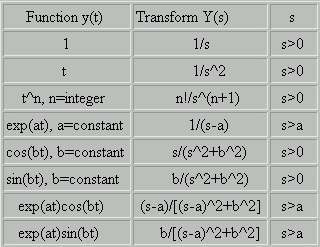As we will show below: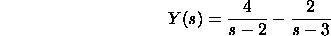Now, we can invert Y(s). From the table, we see that the inverse of
1/(s-2) is exp(2t) and that inverse of 1/(s-3) is exp(3t). Using the
linearity of the inverse transform, we have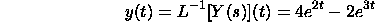The method of partial fractions is a technique for decomposing functions
like Y(s) above so that the inverse transform can be determined in a
straightforward manner. It is applicable to functions of the form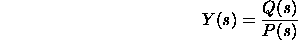where Q(s) and P(s) are polynomials and the degree of Q is less than
the degree of P
. For simplicity we assume that Q and P have real
coefficients. We consider the following cases:

P(s) is a Quadratic with 2 Real RootsThe denominator can be factored: s^2-5s+6=(s-2)(s-3). The denominator
has 2 real roots. In this case we can write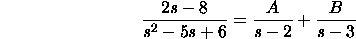Here A and B are numbers. This is always possible if the numerator is
of degree 1 or is a constant. The goal now is to find A and B. Once we
find A and B, we can invert the Laplace transform: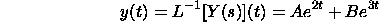If we add the two terms on the RHS, we get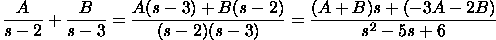Comparing the LHS and RHS, we have: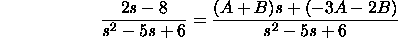The denominators are equal. The two sides will be equal if the numerators
are equal. The numerators are equal for all s if the coefficients of s and the
constant term are equal. Hence, we get the following equations: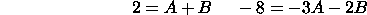This is a system of 2 linear equations with 2 unknowns. It can be
solved by standard methods. We obtain A=4 and B=-2. HenceIf the denominator has n distinct roots: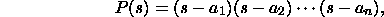then the decomposition has the form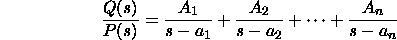where A_1, A_2, ..., A_n are unknown numbers. The inverse
transform is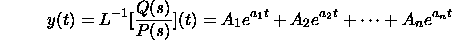The unknown coefficients can be determined using the same
technique as in the case of only 2 factors, as shown above.

P(s) is a Quadratic with a Double Root

Consider the example: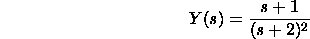The denominator has double root -2. The appropriate decomposition
in this case is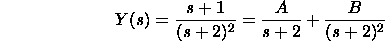Here A and B are numbers. From the table the inverse transform
of 1/(s+2) is exp(-2t) and the inverse transform of 1/(s+2)^2 is
texp(-2t). Hence, the inverse transform of Y(s) is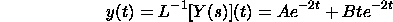Using the same technique as in the case of distinct roots above,
it can be shown that A=1 and B=-2.

P(s) is a Quadratic and has Complex Conjugate Roots

Consider the example: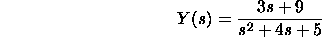The roots of the denominator are -2+/-i. We can complete the
square for the denominator. We have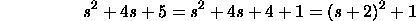Hence, we have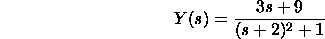Note the denominator (s+2)^2+1 is similar to that for Laplace
transforms of exp(-2t)cos(t) and exp(-2t)sin(t). We need to
manipulate the numerator. Note that in the formula in the table,
we have a=-2 and b=1. We look for a decomposition of the form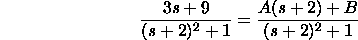If we can find A and B, then: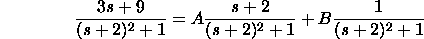The inverse transform is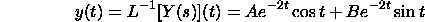We can determine A and B by equating numerators in the expression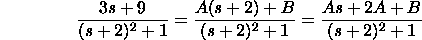Comparing coefficients of s in the numerator we conclude 3=A.
Comparing the constant terms we conclude 2A+B=9. Hence
A=3 and B=3.

P(s) is of Degree 3 and has 1 Real Root and 2 Complex Conjugate Roots

Consider the example: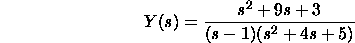The roots of the denominator are 1 and -2+/-i. In this case we
look for a decomposition of the form: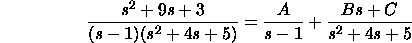where A, B and C are unknowns. The inverse transform of A/(s-1)
is Aexp(t). Once B and C are determined the second term can be
manipulated as in the previous example.